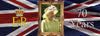# Shape Angles

If you want to know more about shape angles you are in the right place! Read the information below, download a free shape angles guide, a free maths angles poster and read our 2D shape blog for more information about 2D shapes.

## Important Angles Vocabulary

An angle is the size of the turn between two sides at a vertex. Angles are measured in degrees using a protractor.

An acute angle is an angle that is larger than 90º but smaller than 180º.

A right angle is 90º. Right angles are formed when two lines that are perpendicular to each intersect. For example, squares have four right angles.

An obtuse angle is an angle that is larger than 90º but smaller than 180º.

A straight angle is an angle that is 180º. A straight angle is a point on a straight line.

A reflex angle is an angle that is larger than 180º but smaller than 360º.

A complete angle is an angle that measures 360º. It is the full turn around a point.

The interior angles are the angles inside the lines of a shape.

The exterior angles are the angles outside the lines of a shape.

## Angles in polygons

The exterior angles in a polygon always equal 360º

The interior angles of a triangle always equal 180º

The interior angles of a quadrilateral always equal 360º

The interior angles of a pentagon always equal 540º

The interior angles of a hexagon always equal 720º

The interior angles of a heptagon always equal 900º

The interior angles of an octagon always equal 1080º

### Formula to work out the interior angles of polygons

The total size of a shape’s interior angles can be worked out using the formula (n - 2) x 180 where n equals the number of sides.

A square has 4 sides so n = 4

(n - 2) x 180

= (4 - 2) x 180

= 2 x 180

= 360º

A hexagon has 6 sides so n = 6

(n - 2) x 180

= (6 - 2) x 180

= 4 x 180

= 720º

A nonagon has 9 sides so n = 9

(n - 2) x 180

= (9 - 2) x 180

= 7 x 180

= 1260º

## When are angles taught in the Maths National Curriculum?

Angles are taught as part of the geometry strands of the maths curriculum. These strands are geometry - position and direction and geometry - properties of shapes

Angles are first taught in year 2 when children:

In Year 3 children are taught to:

All of these objectives are taught in our ready-to-teach Shapes and Angles scheme of work.

In Year 4 children learn to:

This objective is taught in our downloadable Shape Angles scheme of work.

In Year 5 children learn to:

In Year 6 children are taught to:

### Related Posts##### Queen Elizabeth II Facts for Kids
Queen Elizabeth II is about to celebrate her Platinum Jubilee, but how much do you know about our sovereign? Read these##### What was Danelaw? KS2 Fact Blog
Danelaw was the name of the area of England that at one time belonged to the Vikings. Read PlanBee's Danelaw KS2 Fact Bl